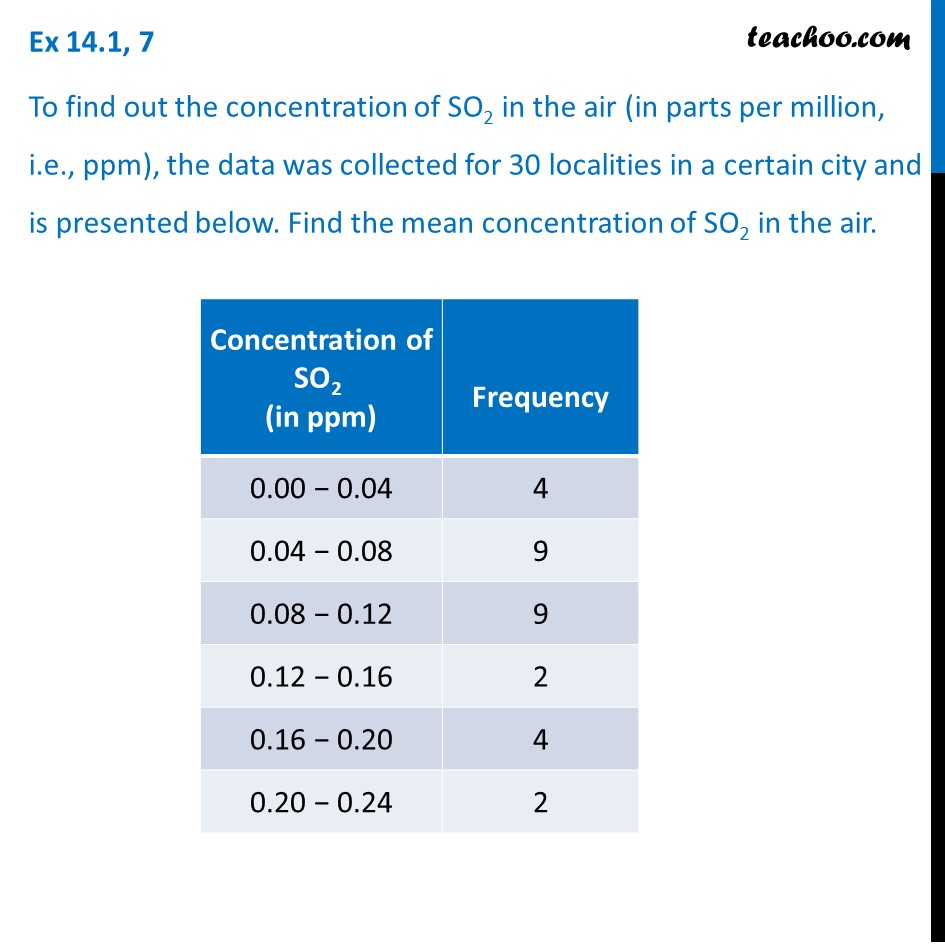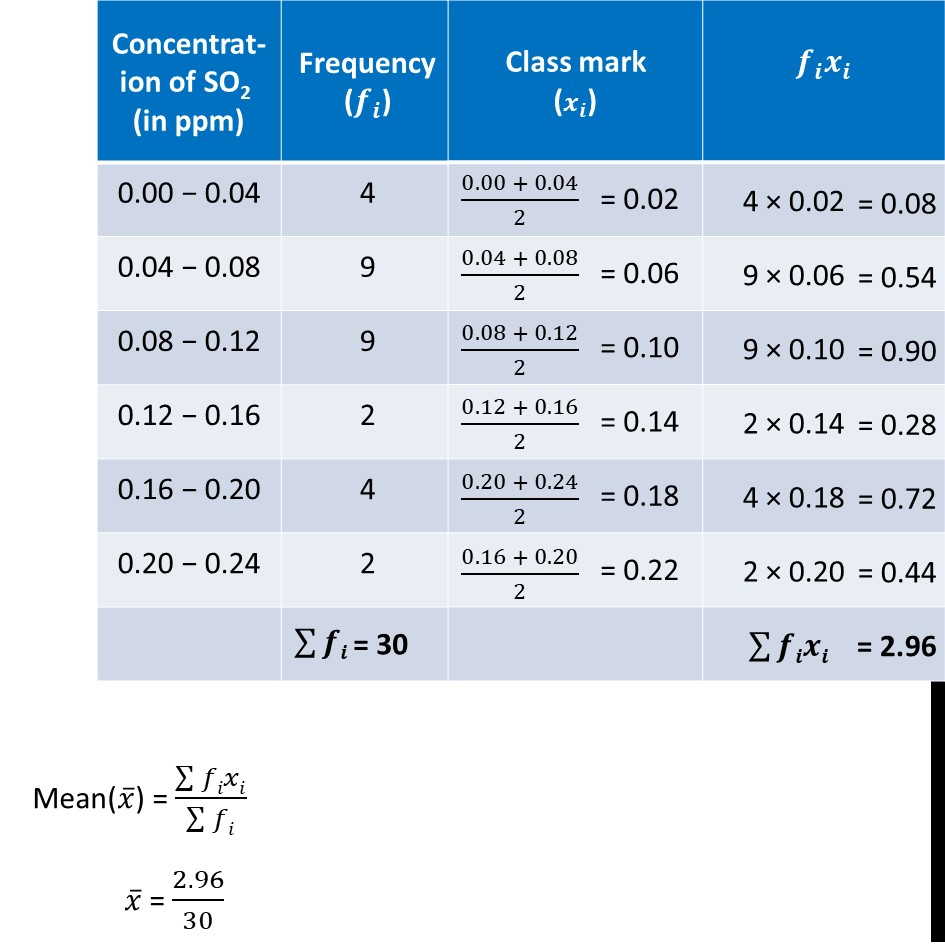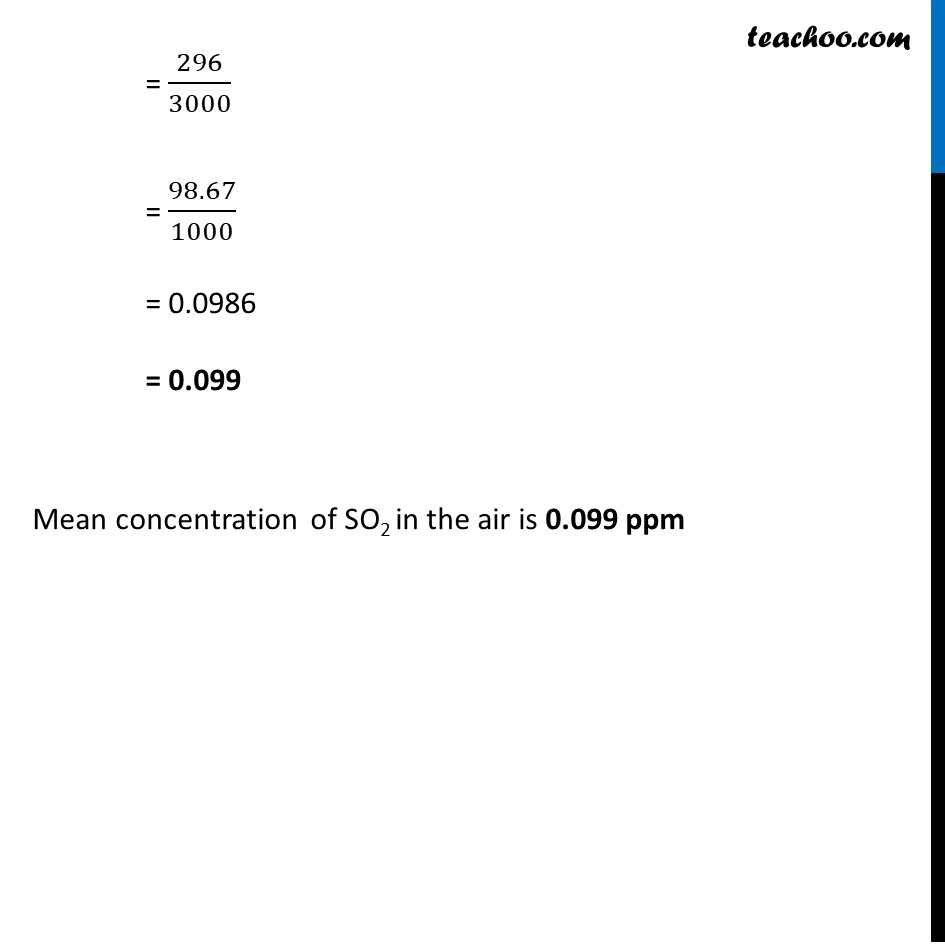1. Chapter 14 Class 10 Statistics (Term 2)
2. Serial order wise
3. Ex 14.1

Transcript

Ex 14.1, 7 To find out the concentration of SO2 in the air (in parts per million, i.e., ppm), the data was collected for 30 localities in a certain city and is presented below. Find the mean concentration of SO2 in the air. Mean(𝑥 ̅) = (∑▒𝑓𝑖𝑥𝑖)/(∑▒𝑓𝑖) 𝑥 ̅ = 2.96/30 = 296/3000 = 98.67/1000 = 0.0986 = 0.099 Mean concentration of SO2 in the air is 0.099 ppm

Ex 14.1